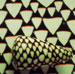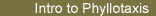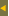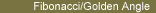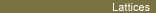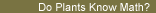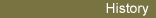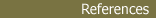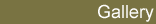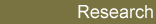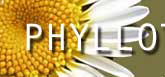## Introduction to Phyllotaxis [Greek phyllo, leaf + taxis, arrangement]

This page presents some of the themes explored in this site. Each theme is linked to a page which opens to an exploration of that theme. For a more leisurely introduction, you can go to the site for our exhibit at the Smith College Botanic Garden.

##### Classification

Most arrangements of leaves fall into 3 or 4 main categories: spiral, distichous, whorled, and multijugate. Spiral arrangements are most frequent and they are classified by the number of spirals (parastichies) they exhibit. Mathematically, all these patterns are types of lattices.##### Fibonacci Numbers - Golden Angle

The number of visible spirals (parastichies) in spiral arrangements are most often Fibonacci numbers (1, 1, 2, 3, 5, 8, 13, 21 ...) and the angle between successive leaves is close to the Golden Angle - about 137.5 degrees. This frequent type of pattern is called Fibonacci phyllotaxis. Fibonacci numbers and the Golden Angle have a precise mathematical relationship.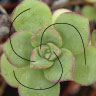##### Microscopic Formation of Plant Patterns

Leaves and other botanical elements initiate as primordia - microscopic bulges of cells at the growing tip of a plant, forming lattice-like patterns.##### Mathematical Model

A simple mathematical model based on the botanist Hofmeister's observations can account for spirals and, more specifically, Fibonacci phyllotaxis. For an in depth view of the mathematics, refer to our Research page.##### So ... Do Plants Know Math? (in construction)

Phyllotaxis has affinities to other pattern formation mechanisms where many simple agents interact with simple rules. What are the evolutionary advantages of Fibonacci phyllotaxis?